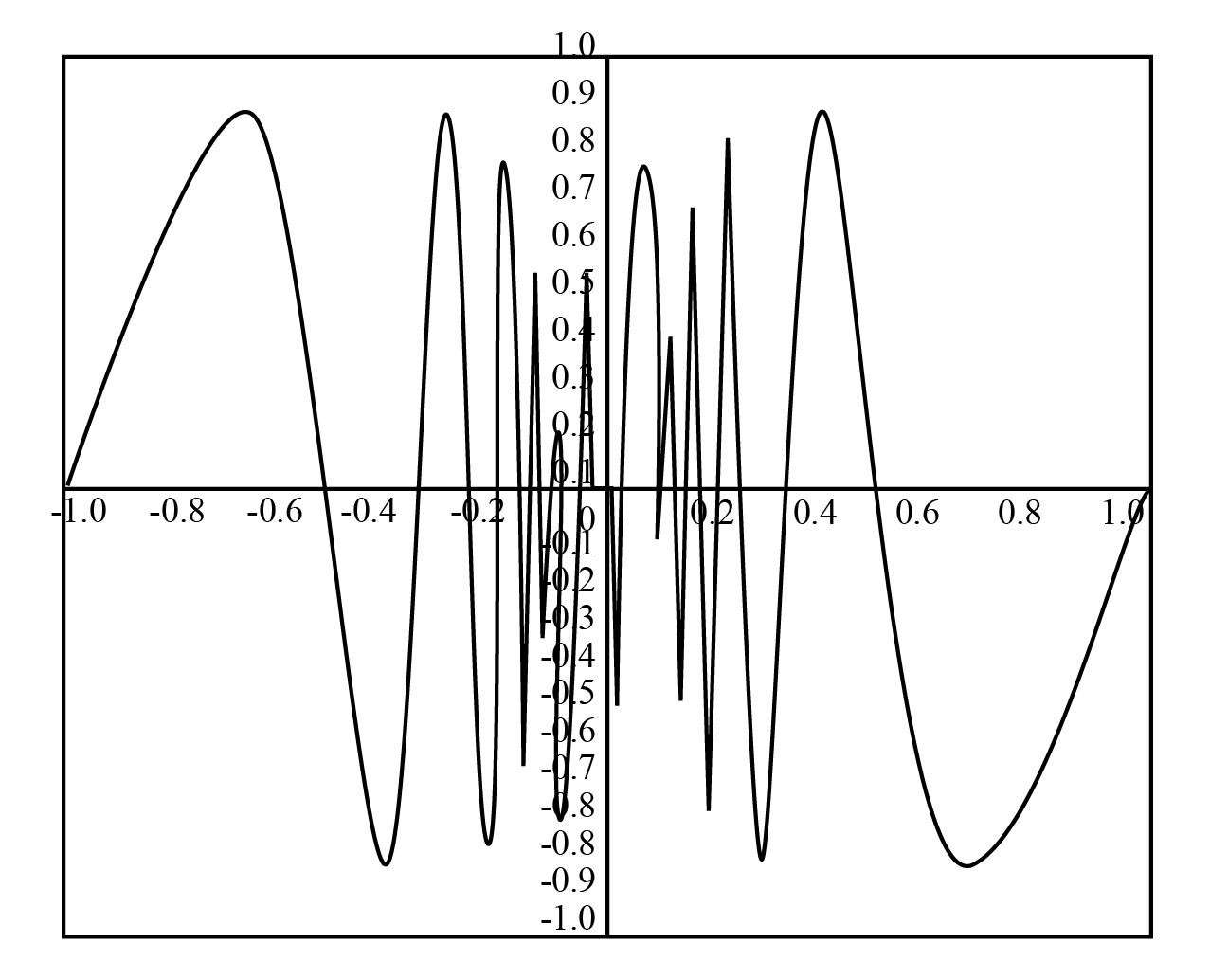×

×

# Graph the function fsxd sinsyxd of Example 4 in the viewing rectangle f21, 1g by f21ISBN: 9781305270336 484

## Solution for problem 51 Chapter 2.2

Single Variable Calculus: Early Transcendentals | 8th Edition

• Textbook Solutions
• 2901 Step-by-step solutions solved by professors and subject experts
• Get 24/7 help from StudySoup virtual teaching assistantsSingle Variable Calculus: Early Transcendentals | 8th Edition

4 5 1 415 Reviews
23
2
Problem 51

Graph the function fsxd sinsyxd of Example 4 in the viewing rectangle f21, 1g by f21, 1g. Then zoom in toward the origin several times. Comment on the behavior of this function.

Step-by-Step Solution:

Problem 51

Graph the functionof Example 4 in the viewing rectangleby. Then zoom in toward the origin several times. Comment on the behaviour of this function.

Step by Step Solution

Step 1 of 3

Graph the functionin the viewing rectanglebyas shown in the figure below:Step 2 of 3

Step 3 of 3

##### ISBN: 9781305270336

The full step-by-step solution to problem: 51 from chapter: 2.2 was answered by , our top Calculus solution expert on 03/19/18, 03:29PM. The answer to “Graph the function fsxd sinsyxd of Example 4 in the viewing rectangle f21, 1g by f21, 1g. Then zoom in toward the origin several times. Comment on the behavior of this function.” is broken down into a number of easy to follow steps, and 32 words. This full solution covers the following key subjects: . This expansive textbook survival guide covers 95 chapters, and 5427 solutions. Since the solution to 51 from 2.2 chapter was answered, more than 223 students have viewed the full step-by-step answer. Single Variable Calculus: Early Transcendentals was written by and is associated to the ISBN: 9781305270336. This textbook survival guide was created for the textbook: Single Variable Calculus: Early Transcendentals, edition: 8.

Unlock Textbook Solution